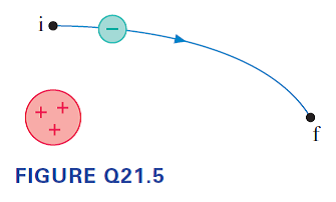# Problem: An electron moves along the trajectory from i to f in Figure Q21.5.a. Does the electric potential energy increase, decrease, or stay the same? Explain.b. Is the electron's speed at f greater than, less than, or equal to its speed at i? Explain.

###### FREE Expert Solution

a.

Electrostatic potential energy:

$\overline{){\mathbf{U}}{\mathbf{=}}\frac{\mathbf{k}{\mathbf{q}}_{\mathbf{1}}{\mathbf{q}}_{\mathbf{2}}}{\mathbf{r}}}$

We have a negative and a positive charge:

96% (122 ratings)###### Problem Details

An electron moves along the trajectory from i to f in Figure Q21.5.a. Does the electric potential energy increase, decrease, or stay the same? Explain.

b. Is the electron's speed at f greater than, less than, or equal to its speed at i? Explain.